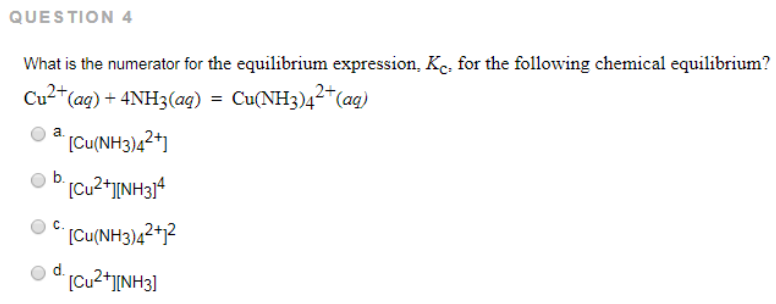# Problem: What is the numerator for the equilibrium expression, Kc, for the following chemical equilibrium? Cu2+ (aq) + 4NH3 (aq) = Cu(NH3)42+ (aq) a. [Cu(NH3)42+]b. [Cu2+][NH3]4 c. [Cu(NH3)42+]2 d. [Cu2+][NH3]

###### FREE Expert Solution
99% (81 ratings)###### Problem Details

What is the numerator for the equilibrium expression, Kc, for the following chemical equilibrium?

Cu2+ (aq) + 4NH3 (aq) = Cu(NH3)42+ (aq)

a. [Cu(NH3)42+]

b. [Cu2+][NH3]4

c. [Cu(NH3)42+]2

d. [Cu2+][NH3]